Talk is cheap , show me your code!

# Java集合之HashMap详解

Map和之前List和Set最大的不同就是，Set和List往里面存元素的时候是以对象的形式存进去的，而map的以映射Key-Value方式存进去的，Map的key是不可变的。

## HashMap

### 基本概念

HashMap是线程不安全的，是基于Key-Value的映射关系存储的键值对。在jdk1.7底层是数组+链表实现的，1.8开始是数组+链表/红黑树实现的，HashMap的默认初始容量是16，两倍扩容，负载因子是0.75，HashMap总是使用2的幂作为哈希表的大小。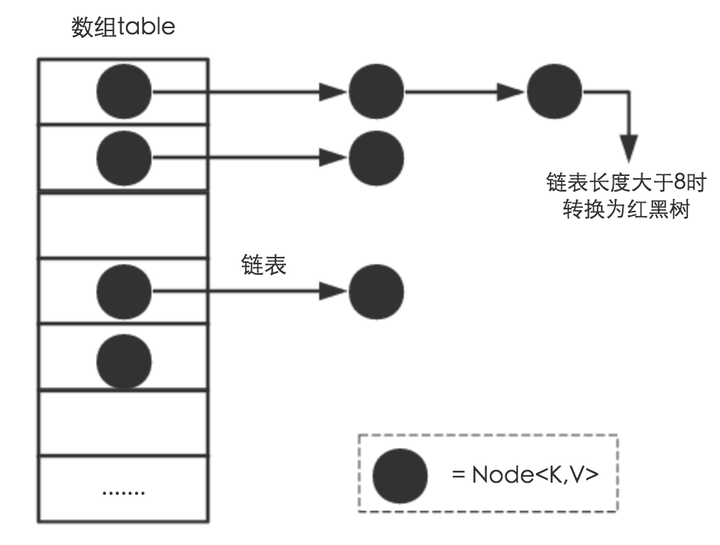HashMap中有一个东西叫做Node，简单解释就是哈希桶数组，每一对key-value都可以称作哈希桶数组，HashMap由很多个哈希桶数组组成，HashMap使用HashTable来存储数据的。
jdk1.8中Node的代码：

``````    static class Node<K,V> implements Map.Entry<K,V> {
final int hash;
final K key;
V value;
Node<K,V> next;

Node(int hash, K key, V value, Node<K,V> next) {
this.hash = hash;
this.key = key;
this.value = value;
this.next = next;
}``````

### HashMap的构造函数

HashMap有无参构造函数和有参构造函数。

``````    public HashMap() {
}``````

``````    public HashMap(int initialCapacity) {
}``````

``````    public HashMap(int initialCapacity, float loadFactor) {
if (initialCapacity < 0)
throw new IllegalArgumentException("Illegal initial capacity: " +
initialCapacity);
if (initialCapacity > MAXIMUM_CAPACITY)
initialCapacity = MAXIMUM_CAPACITY;
throw new IllegalArgumentException("Illegal load factor: " +
this.threshold = tableSizeFor(initialCapacity);
}``````

``````    /**
* Returns a power of two size for the given target capacity.
*/
static final int tableSizeFor(int cap) {
int n = cap - 1;
n |= n >>> 1;
n |= n >>> 2;
n |= n >>> 4;
n |= n >>> 8;
n |= n >>> 16;
return (n < 0) ? 1 : (n >= MAXIMUM_CAPACITY) ? MAXIMUM_CAPACITY : n + 1;
}``````

1.为什么要将初始容量-1呢？

• 第一次右移1位，0100，在和原来的1000做或操作，结果为1100；
• 第二次右移2位，0011，或操作，1111；
• 第三次右移4位，0000，或操作，1111；
• 第四次右移8位，0000，或操作，1111；
• 第五次右移16位，0000，或操作，1111；

2.五次右移+或操作，+1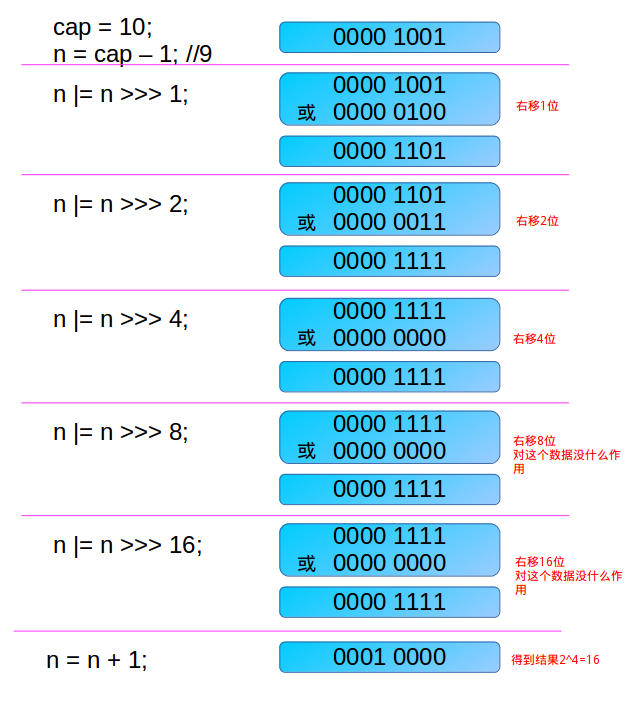10的二进制表示为1001，经过-1和五次右移+或操作后在+1，结果为10000，也就是16，大于等于10的最小的2的幂正好也是16。

8的二进制表现形式为1000，-1之后为0111，

• 第一次右移1位，0011，在和原来的0111做或操作，结果为0111；
• 第二次右移2位，0001，或操作，0111；
• 第三次右移4位，0001，或操作，0111；
• 第四次右移8位，0001，或操作，0111；
• 第五次右移16位，0001，或操作，0111；

### HashMap是如何计算数组下标索引的？

1.7Hash算法

``````        static int hash(int h) {
h ^= (h >>> 20) ^ (h >>> 12);
return h ^ (h >>> 7) ^ (h >>> 4);
}``````

``````    static final int hash(Object key) {
int h;
return (key == null) ? 0 : (h = key.hashCode()) ^ (h >>> 16);
}``````

key调用HashCode方法得到HashCode值，然后异或哈希码右移16的结果，换个思路说就是高16位和低16位进行异或操作。

[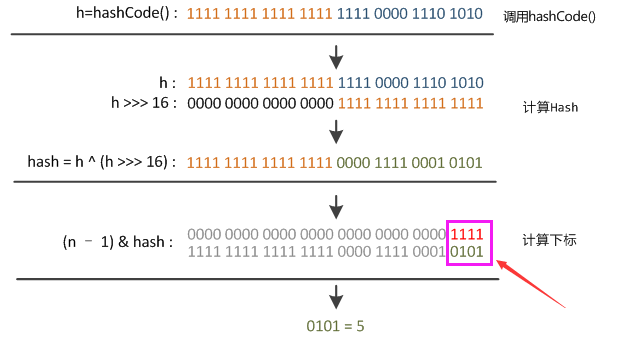]

### 1.7与1.8 HashMap的put方法与扩容机制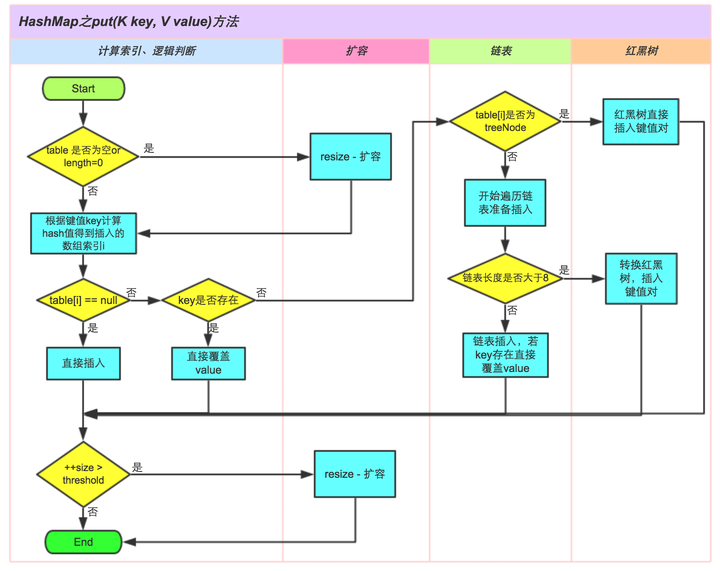#### 1.7的put方法，扩容机制以及造成死锁的原因

``````public V put(K key, V value)
{
......
//算Hash值
int hash = hash(key.hashCode());
//计算数组下标索引
int i = indexFor(hash, table.length);
//如果该key已被插入，则替换掉旧的value （链接操作）
for (Entry<K,V> e = table[i]; e != null; e = e.next) {
Object k;
if (e.hash == hash && ((k = e.key) == key || key.equals(k))) {
V oldValue = e.value;
e.value = value;
e.recordAccess(this);
return oldValue;
}
}
modCount++;
//该key不存在，需要增加一个结点
return null;
}``````

``````void addEntry(int hash, K key, V value, int bucketIndex)
{
Entry<K,V> e = table[bucketIndex];
table[bucketIndex] = new Entry<K,V>(hash, key, value, e);
//查看当前的size是否超过了我们设定的阈值threshold，如果超过，需要resize
if (size++ >= threshold)
resize(2 * table.length);
} ``````

``````void resize(int newCapacity)
{
Entry[] oldTable = table;
int oldCapacity = oldTable.length;
......
//创建一个新的Hash Table
Entry[] newTable = new Entry[newCapacity];
//将Old Hash Table上的数据迁移到New Hash Table上
transfer(newTable);
table = newTable;
}``````

``````void transfer(Entry[] newTable)
{
Entry[] src = table;
int newCapacity = newTable.length;
//下面这段代码的意思是：
//  从OldTable里摘一个元素出来，然后放到NewTable中
for (int j = 0; j < src.length; j++) {
Entry<K,V> e = src[j];
if (e != null) {
src[j] = null;
do {
Entry<K,V> next = e.next;
int i = indexFor(e.hash, newCapacity);
e.next = newTable[i];
newTable[i] = e;
e = next;
} while (e != null);
}
}
} ``````

#### HashMap 1.7潜在的安全隐患

String的HashCode方法是公开的，如果有人通过精心构造大量的的hashcode相同字符串使哈希表每次都产生碰撞，该数组解决哈希碰撞的链表就会非常长，以至于最后会退化成链表，链表的查询速度是很低的，这样就会能造成hash碰撞攻击，消耗大量CPU资源，导致系统无法快速响应请求，从而达到拒绝服务攻击（DoS）的目的。

### 1.8HashMap的改进

#### 数组+链表/红黑树

` static final int TREEIFY_THRESHOLD = 8;`
` static final int MIN_TREEIFY_CAPACITY = 64;`

``````     * 0:    0.60653066
* 1:    0.30326533
* 2:    0.07581633
* 3:    0.01263606
* 4:    0.00157952
* 5:    0.00015795
* 6:    0.00001316
* 7:    0.00000094
* 8:    0.00000006
* more: less than 1 in ten million``````

#### 扩容插入顺序

`````` /**
* Implements Map.put and related methods
*
* @param hash hash for key
* @param key the key
* @param value the value to put
* @param onlyIfAbsent if true, don't change existing value
* @param evict if false, the table is in creation mode.
* @return previous value, or null if none
*/
final V putVal(int hash, K key, V value, boolean onlyIfAbsent,
boolean evict) {
Node<K,V>[] tab; Node<K,V> p; int n, i;
if ((tab = table) == null || (n = tab.length) == 0)
n = (tab = resize()).length;
if ((p = tab[i = (n - 1) & hash]) == null)
tab[i] = newNode(hash, key, value, null);
else {
Node<K,V> e; K k;
if (p.hash == hash &&
((k = p.key) == key || (key != null && key.equals(k))))
e = p;
else if (p instanceof TreeNode)
e = ((TreeNode<K,V>)p).putTreeVal(this, tab, hash, key, value);
else {
for (int binCount = 0; ; ++binCount) {
if ((e = p.next) == null) {
p.next = newNode(hash, key, value, null);
if (binCount >= TREEIFY_THRESHOLD - 1) // -1 for 1st
treeifyBin(tab, hash);
break;
}
if (e.hash == hash &&
((k = e.key) == key || (key != null && key.equals(k))))
break;
p = e;
}
}
if (e != null) { // existing mapping for key
V oldValue = e.value;
if (!onlyIfAbsent || oldValue == null)
e.value = value;
afterNodeAccess(e);
return oldValue;
}
}
++modCount;
if (++size > threshold)
resize();
afterNodeInsertion(evict);
return null;
}``````

``````/**
* Initializes or doubles table size.  If null, allocates in
* accord with initial capacity target held in field threshold.
* Otherwise, because we are using power-of-two expansion, the
* elements from each bin must either stay at same index, or move
* with a power of two offset in the new table.
*
* @return the table
*/
final Node<K,V>[] resize() {
Node<K,V>[] oldTab = table;
int oldCap = (oldTab == null) ? 0 : oldTab.length;
int oldThr = threshold;
int newCap, newThr = 0;
...
if (oldTab != null) {
for (int j = 0; j < oldCap; ++j) {
Node<K,V> e;
if ((e = oldTab[j]) != null) {
oldTab[j] = null;
if (e.next == null)
newTab[e.hash & (newCap - 1)] = e;
else if (e instanceof TreeNode)
((TreeNode<K,V>)e).split(this, newTab, j, oldCap);
else { // preserve order
Node<K,V> loHead = null, loTail = null;
Node<K,V> hiHead = null, hiTail = null;
Node<K,V> next;
do {
next = e.next;
if ((e.hash & oldCap) == 0) {
if (loTail == null)
else
loTail.next = e;
loTail = e;
}
else {
if (hiTail == null)
else
hiTail.next = e;
hiTail = e;
}
} while ((e = next) != null);
if (loTail != null) {
loTail.next = null;
}
if (hiTail != null) {
hiTail.next = null;
}
}
}
}
}
return newTab;
}``````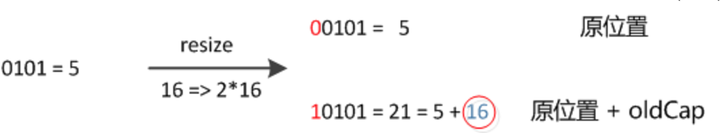## 参考资料

### 觉得文章有用就打赏一下文章作者

#### 支付宝扫一扫打赏#### 微信扫一扫打赏• QQ交流
• 回顶